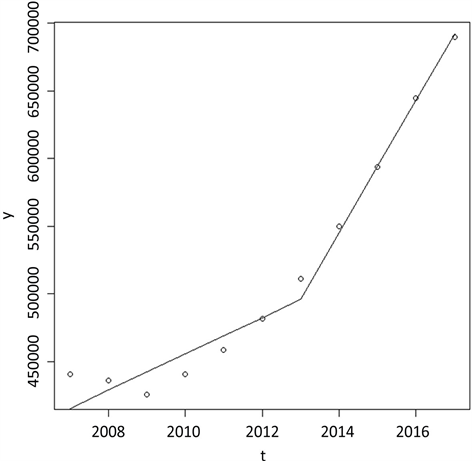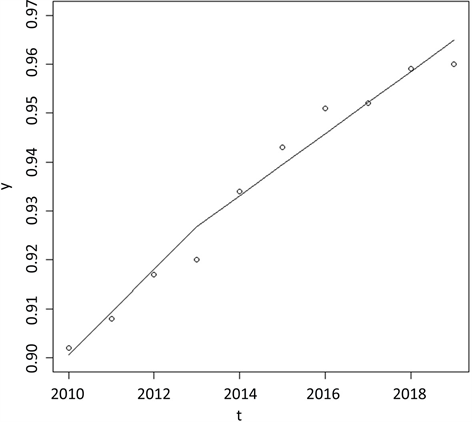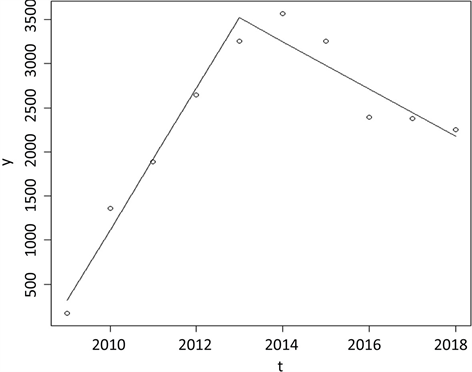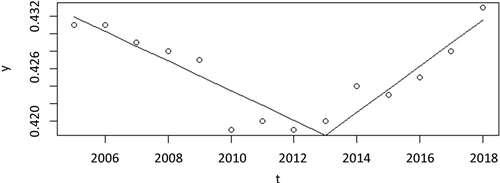# 塑料瓶装水禁令影响力的定量评估Quantitative Evaluation of the Influence of Ban on Plastic Bottled Water

DOI: 10.12677/AAM.2020.92025, PDF, HTML, XML, 下载: 122  浏览: 223

Abstract: Since 2013, the ban on plastic bottled water has been passed and enforced in many parts of the United States. Proponents of the ban believe that growing plastic products produce a large amount of plastic waste, which has brought serious environmental harm to the earth. Opponents of the ban believe that plastic bottled water is a necessary product in crisis, and its importance is self-evident. They believe that the ban will also cause unemployment in related industries. In this paper, a piecewise linear robust regression model is used to model environmental and economic indicators, and a measure of the impact of plastic bottled water ban on a single indicator is given. On this basis, AHP is used to determine the weight of environmental and economic indicators, so as to give a quantitative evaluation of the comprehensive impact of the ban on plastic bottled water.

1. 引言

2013年以来，塑料瓶装水禁令在美国的许多地方被通过并执行，其中包括马塞诸塞州的康科德镇，加州的旧金山和其他一些西方国家的城市。根据政府颁布的相关法令，体积小于1升的塑料瓶装饮用水的售卖被禁止，但其他饮料和更大包装饮用水的售卖不受限制。禁令的支持者认为日益增长的塑料制品需求给地球带来了严重的危害，塑料瓶装水不仅对水源地的环境有负面影响，在生产中也消耗大量的能量及资源，同时还产生大量不可降解废料，造成多种环境污染问题。但禁令的反对者认为，在危机情况下，比如地震、飓风时，人们无法通过其他方式获取可饮用的水，塑料瓶装水相当地重要；他们还对此禁令在相关工业中可能造成的失业问题表示担忧。

2. 塑料瓶装水禁令对单指标的影响力模型

2.1. 分段线性回归模型引入

2013年是美国瓶装水禁令的关键时间点。我们认为该禁令对经济，环境均有影响。如塑料瓶装水的放弃PET作为塑料瓶装水的材料。为此，我们认为，塑料瓶装水的销售将直接受到该禁令影响，进而影响公司的营业和产品。我们进一步认为，瓶装水销量的增速会放缓，这会导致公司量和PET公司的收入来衡量禁令的影响。而塑料瓶装水销量减少也可能造成塑料类垃圾的减少。

2.2. 分段线性稳健回归模型与求解

${D}_{{t}_{0}}\left(t\right)=\left\{\begin{array}{l}1\text{ }\text{ }t\ge {t}_{0}\\ 0\text{ }t<{t}_{0}\end{array}$ (1)

$y\left(t\right)={\beta }_{0}+{\beta }_{1}t+{\beta }_{2}\left(t-{t}_{0}\right){D}_{{t}_{0}}\left(t\right)+\epsilon \left(t\right)$ (2)

$\begin{array}{c}E\left[y\left(t\right)\right]={\beta }_{0}+{\beta }_{1}t+{\beta }_{2}\left(t-{t}_{0}\right){D}_{{t}_{0}}\left(t\right)\\ =\left\{\begin{array}{l}{\beta }_{0}+{\beta }_{1}t\text{ }\text{ }\text{ }\text{ }\text{ }\text{\hspace{0.17em}}\text{ }\text{\hspace{0.17em}}\text{\hspace{0.17em}}\text{ }\text{ }t<{t}_{0}\\ {\beta }_{0}-{\beta }_{2}{t}_{0}+\left({\beta }_{1}+{\beta }_{2}\right)t\text{ }\text{\hspace{0.17em}}\text{\hspace{0.17em}}\text{ }t\ge {t}_{0}\end{array}\end{array}$ (3)

$l\left({\beta }_{0},{\beta }_{1},{\beta }_{2}\right)=\underset{i=1}{\overset{n}{\sum }}|{y}_{i}-{\beta }_{0}-{\beta }_{1}{t}_{i}-{\beta }_{2}\left({t}_{i}-{t}_{0}\right){D}_{{t}_{0}}\left({t}_{i}\right)|$ (4)

$l\left({\stackrel{^}{\beta }}_{0},{\stackrel{^}{\beta }}_{1},{\stackrel{^}{\beta }}_{2}\right)=\underset{{\beta }_{0},{\beta }_{1},{\beta }_{2}\in R}{\mathrm{min}}l\left({\beta }_{0},{\beta }_{1},{\beta }_{2}\right)=\underset{{\beta }_{0},{\beta }_{1},{\beta }_{2}\in R}{\mathrm{min}}\underset{i=1}{\overset{n}{\sum }}|{y}_{i}-{\beta }_{0}-{\beta }_{1}{t}_{i}-{\beta }_{2}\left({t}_{i}-{t}_{0}\right){D}_{{t}_{0}}\left({t}_{i}\right)|$ (5)

2.3. 塑料瓶装水禁令对单指标影响力的度量

$y\left(t\right)={\beta }_{0}+{\beta }_{1}t+{\beta }_{2}\left(t-{t}_{0}\right){D}_{{t}_{0}}\left(t\right)+\epsilon \left(t\right)$ (6)

${I}_{{t}_{0}}\left(T\right)=\frac{\underset{t={t}_{0}}{\overset{{t}_{0}+T}{\sum }}{\beta }_{2}\left(t-{t}_{0}\right)}{\underset{t={t}_{0}}{\overset{{t}_{0}+T}{\sum }}\left({\beta }_{0}+{\beta }_{1}t\right)}$ (7)

${I}_{{t}_{0}}\left(T\right)$ 的分子 $\underset{t={t}_{0}}{\overset{{t}_{0}+T}{\sum }}{\beta }_{2}\left(t-{t}_{0}\right)=\underset{t={t}_{0}}{\overset{{t}_{0}+T}{\sum }}\left[\left({\beta }_{0}+{\beta }_{1}t+{\beta }_{2}\left(t-{t}_{0}\right)\right)-{\beta }_{0}+{\beta }_{1}t\right]$ 表示在时刻 ${t}_{0}$ 后T时间内由塑料瓶禁令的影响指标值增加量之和，分母表示在时刻 ${t}_{0}$ 后T时间内不受塑料瓶禁令的影响指标值之和，因此 ${I}_{{t}_{0}}\left(T\right)$ 表示在时刻 ${t}_{0}$ 后T时间内由于塑料瓶禁令影响相对变化的量。如果 ${I}_{{t}_{0}}\left(T\right)>0$，则 ${I}_{{t}_{0}}\left(T\right)$ 表示在时刻 ${t}_{0}$ 后T时间内由于塑料瓶禁令影响相对增加的比例；如果 ${I}_{{t}_{0}}\left(T\right)<0$，则 ${I}_{{t}_{0}}\left(T\right)$ 表示在时刻 ${t}_{0}$ 后T时间内由于塑料瓶禁令影响相对减少的比例。

${I}_{{t}_{0}}\left(T\right)=\frac{\underset{t={t}_{0}}{\overset{{t}_{0}+T}{\sum }}{\beta }_{2}\left(t-{t}_{0}\right)}{\underset{t={t}_{0}}{\overset{{t}_{0}+T}{\sum }}\left({\beta }_{0}+{\beta }_{1}t\right)}=\frac{{\beta }_{2}\frac{T\left(T+1\right)}{2}}{{\beta }_{0}\left(T+1\right)+{\beta }_{1}\frac{T\left(T+1\right)}{2}}$ (8)

$I=\frac{{\beta }_{2}}{{\beta }_{1}}$

3. 塑料瓶装水禁令对美国环境、经济等影响力的度量

3.1. 塑料瓶装水禁令对PET塑料瓶装水垃圾量的影响力度量Table 1. Total waste amount of pet plastic bottled water in the United States, 2007~2017 (Unit: m3)

$y\left(t\right)=402081+13406t+35671\left(t-7\right){D}_{7}\left(t\right)=\left\{\begin{array}{l}402081+13406t,\text{ }t<7\\ 152380+49077t,\text{ }t\ge 7\end{array}$Figure 1. Piecewise linear robust regression fitting curve of total waste amount of pet plastic bottled water, 2007~2017

${I}_{7}\left(4\right)=0.14$

$I=\frac{49077}{13406}=3.66$

3.2. 塑料瓶装水禁令对就业率的影响力度量Table 2. Rate of employment in the United States, 2010~2019

$y\left(t\right)=0.8920+0.0087t-0.0024\left(t-4\right){D}_{4}\left(t\right)=\left\{\begin{array}{l}0.8920+0.0087t,\text{ }t<4\\ 0.9016+0.0063t,\text{ }t\ge 4\end{array}$Figure 2. Piecewise linear robust regression fitting curve of the rate of employment in the United States, 2010~2019

${I}_{4}\left(7\right)=-0.0076$

$I=-\frac{0.0024}{0.0087}=-0.2759$

3.3. 塑料瓶禁令对PET公司收入影响力度量

Lyondell Basell是一家PET塑料公司，我们收集了公司的2009~2018年收入的数据，见表3Table 3. Annual income of the Lyondell Baselld, 2009~2018 (Unit: Million USD)

$y\left(t\right)=142.2+468.2t-266.2\left(t-5\right){D}_{5}\left(t\right)=\left\{\begin{array}{l}142.2+468.2t,\text{ }t<5\\ 1475.2+201.6t,\text{\hspace{0.17em}}\text{\hspace{0.17em}}t\ge 5\end{array}$Figure 3. Piecewise linear robust regression fitting curve of the gross profit of Lyondell Basell

${I}_{5}\left(5\right)=-0.18$

$I=-\frac{266.6}{468.2}=-0.57$

3.4. 塑料瓶禁令对可口可乐市场占有率影响力度量Table 4. Coca Cola’s market share, 2004~2018

$y\left(t\right)=0.4337-0.0017t+\text{0}\text{.0043}\left(t-5\right){D}_{9}\left(t\right)=\left\{\begin{array}{l}0.4337-0.0017t,\text{ }t<9\\ \text{0}\text{.4122}+\text{0}\text{.0026,}\text{ }\text{\hspace{0.17em}}t\ge 9\end{array}$Figure 4. Piecewise linear robust regression fitting curve of the Coca Cola’s market share, 2005~2018

${I}_{9}\left(5\right)=2.5\text{%}$

$I=\text{2}\text{.53}$

4. 塑料瓶装水禁令综合影响力的定量评估

4.1. 构造判断矩阵

${a}_{ij}>0,{a}_{ij}=\frac{1}{{a}_{ji}},i,j=1,2,\cdots ,n$

${a}_{ij}{a}_{jk}={a}_{ik},i,j,k=1,2,\cdots ,n$Table 5. Satty 9 scale meaning table

$A=\left[\begin{array}{cccc}1& 1/2& 4& 3\\ 2& 1& 6& 5\\ 1/4& 1/4& 1& 1/3\\ 1/3& 1/5& 3& 1\end{array}\right]$

4.2. 计算权重排序向量与一致性检验

${\lambda }_{\mathrm{max}}=4.15,w={\left(\text{0}\text{.338,0}\text{.407,0}\text{.095,0}\text{.160}\right)}^{\text{T}}$

1) 计算一致性指标 $C.I.$

$C.I.=\frac{{\lambda }_{\mathrm{max}}-n}{n-1}=\frac{4.15-4}{4-1}=0.05$

2) 查找相应的平均随机一致性指标 $R.I.$，下表给出了1~11阶正互反矩阵的平均随机一致性指标。

3) 计算一致性比例 $C.R.=\frac{C.I.}{R.I.}$。当 $C.R.<0.10$ 时，认为判断矩阵的一致性是可以接受的，否则应对判断矩阵作适当修正。

$C.R.=\frac{C.I.}{R.I.}=\frac{0.05}{0.9}=0.055<0.10$

4.3. 塑料瓶装水禁令的长期综合影响力度量

$-3.66{w}_{1}-0.2759{w}_{2}-0.57{w}_{3}+2.53{w}_{4}=-0.9987$Table 6. Measurement of the Influence of Prohibition on relevant Indicators

5.模型的优缺点

5.1. 模型的优点

5.2. 模型的缺点

  王黎明, 陈颖, 杨楠. 应用回归分析[M]. 上海: 复旦大学出版社, 2014: 164-153.  韩中庚. 数学建模方法及其应用[M]. 第7版. 北京: 高等教育出版社, 2017: 136-141.